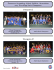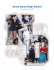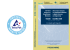# Computational modeling of multiscale flows - C-SWARM

## Transcription

Computational modeling of multiscale flows - C-SWARM
```Computational modeling of
multiscale flows
T. Grenga, S. Paolucci
Department of Aerospace & Mechanical Engineering
Center for Shock Wave-processing
(C-SWARM)
WCCM XI - ECCM V - ECFD VI - Barcelona 21 July 2014
Motivation
“a single overarching grand challenge: the development of a validated,
predictive, multi-scale, combustion modeling capability to optimize the
design and operation of evolving fuels in advanced engines”,
Basic Research Needs for Clean and Efficient Combustion of 21-st
Century Transportation Fuels, DOE 2007
Models need to simulate the intricate coupling between
the fluid mechanics and chemistry
Multi-scales problems require
• high performance computing
T. Grenga, S. Paolucci — University of Notre Dame (C-SWARM) — Computational modeling of multiscale flows
2
Introduction
The model includes detailed
chemical kinetics, multicomponent diffusion
Problems are typically
multidimensional and contain
a wide range of spatial and
temporal scales
to the simulation of
“Research needs for future internal
combustion engines”,
Physics Today, Nov. 2008, pp. 47-52
The method resolves the range of spatial scales
present, while greatly reducing computational cost
T. Grenga, S. Paolucci — University of Notre Dame (C-SWARM) — Computational modeling of multiscale flows
3
Governing Equations
∂ρ
∂t
∂ρui
∂t
∂ρE
∂t
∂ρYk
∂t
∂
=−
(ρui )
∂xi
∂
∂τij
=−
(ρuj ui + pδij ) +
∂xj
∂xj
∂
∂
=−
(uj (ρE + p)) +
(uj τji − qi )
∂xj
∂xi
∂
∂ji,k
=−
(ui ρYk ) −
+ Mk ω̇k ,
k = 1, . . . , K − 1
∂xi
∂xi
ρ-density, ui -velocity vector, p-pressure, E-specific total energy, Yk -mass fraction of species k, τij -viscous stress tensor, qi -heat flux, ji,k -species mass flux,
Mk - molecular mass of species k, and ω̇k -production rate of species k
T. Grenga, S. Paolucci — University of Notre Dame (C-SWARM) — Computational modeling of multiscale flows
4
Governing Equations
�
�
�
�
∂ul
∂ui
∂uj
2
τij = µ
+
δij
+ κ− µ
∂xj
∂xi
3
∂xl
�
K �
�
∂T
RT
hk ji,k −
qi = −λ
+
DTk di,k
∂xi
Mk X k
k=1
ji,k
ρYk
=
M Xk
K
�
j=1
j�=k
Mj Dkj di,j
DTk ∂T
−
T ∂xi
k=1
∂Xk
1 ∂p
di,k =
+ (Xk − Yk )
∂xi
p ∂xi
�
�
K
I
K
�
� � ��
�
�
�
��
�
f
νki
r
νki
νki − νki
ki
ω̇k =
[Xk ] − ki
[Xk ]
i=1
1
E = e + ui ui
2
ρRT
p=
M
K
�
Yk = 1
k=1
k=1
e-internal energy, T -temperature, R-universal gas constant, M -mixture molecular mass, µ-shear viscosity, κ-bulk viscosity, λ-thermal conductivity, hk -enthalpy
of species k, Xk -mole fraction of species k, di,k -diﬀusion driving force, DkT thermal diﬀusion coeﬃcients, Dij -ordinary multicomponent diﬀusion coeﬃcients,
�
��
νki and νki -product and reactant stoichiometric coeﬃcients in reaction i, kif and
kir forward and reverse reaction rate constants
T. Grenga, S. Paolucci — University of Notre Dame (C-SWARM) — Computational modeling of multiscale flows
5
Representation (WAMR) Method
l - l0
It is a dynamically spatially
6
Collocation points are
4
associated with wavelets
2
Wavelet amplitudes reflect the
local regularity of functions
0
1.0
High resolution grid with small
0.5
number of points
y
Solutions are automatically verified
Method is parallelized with MPI approach
1.0
0.5
0.0 0.0
x
S. Paolucci, Z. Zikoski, D. Wiraseat, WAMR: An adaptive method for the simulation of compressible
reacting flow. Part I. Accuracy and efficiency of algorithm, J. Comp. Phys., 272 (2014), pp. 814-841
S. Paolucci, Z. Zikoski, T. Grenga, WAMR: An adaptive method for the simulation of compressible
reacting flow. Part II. The parallel algorithm, J. Comp. Phys., 272 (2014), pp. 842-864
T. Grenga, S. Paolucci — University of Notre Dame (C-SWARM) — Computational modeling of multiscale flows
6
WAMR Method
Sparse wavelet representation (SWR)
u (x) =
J
�
�
u0,k Φ0,k (x) +
J−1
�
�
dj,λ Ψj,λ (x)
j=0 {λ : |dj,λ |≥ε}
k
��
�
uJ
ε
��
�
RεJ
Density
Pressure
Temperature
order 1/6
−2
−d/p
Max error
−3
10
(i) J
Dx uε �V,∞
1
−4
10
−5
10
−6
10
Error of derivative approximation
�∂ u/∂x −
dj,λ Ψj,λ (x),
10
Number of collocation points
i
0
−1
�u − uJε �∞ ≤ C1 ε
i
�
10
Error in SWR:
�
j=0 {λ : |dj,λ |<ε}
10
Threshold parameter: ε
NE ≤ C2 ε
+
J−1
�
≤
−6
10
−5
10
−4
10
−3
ε
10
−2
10
−1
10
− min((p−i),n)/2
CNE
T. Grenga, S. Paolucci — University of Notre Dame (C-SWARM) — Computational modeling of multiscale flows
7
WAMR: 3D Shock-Bubble interaction (SBI)
Problem Description
• Domain
[0, 3]×[0, 0.4]×[0, 0.4] cm
• Ambient Mixture
YN2=0.868, YO2=0.232
P=101.3 kPa
T=1000 K
• Bubble
YH2=0.990
r=0.1 cm at x=0.5cm
Ms = 1.5 at x=0.3 cm
• Wavelet parameter
ε=10-2
p=4
[Nx× Ny × Nz]coarse=[60×8×8]
J=6
Δxmin= 7.81 µm
• Time integration
Runge-Kutta-Fehlberg 45
εrel=10-3, εabs=10-10
T. Grenga, S. Paolucci — University of Notre Dame (C-SWARM) — Computational modeling of multiscale flows
8
WAMR: 3D SBI - Results
3
Density [g/cm ]
Temperature [K]
1000
1100
1200
1300
1400
1500
4.0e-4
1600
5.0e-4
6.0e-4
7.0e-4
8.0e-4
0µs
2.5µs
5µs
10µs
18.75µs
T. Grenga, S. Paolucci — University of Notre Dame (C-SWARM) — Computational modeling of multiscale flows
9
WAMR: 3D SBI - Results
HO2
Velocity [cm/s]
20000
40000
60000
80000
4.0e-5
100000
6.0e-5
8.0e-5
1.0e-4
1.2e-4
0µs
2.5µs
5µs
10µs
18.75µs
T. Grenga, S. Paolucci — University of Notre Dame (C-SWARM) — Computational modeling of multiscale flows
10
WAMR: 3D SBI - Results 10μs
H2
5.00e-02
4.00e-02
3.00e-02
2.00e-02
1.00e-02
H2O2
1.60e-08
1.20e-08
8.00e-09
4.00e-09
T. Grenga, S. Paolucci — University of Notre Dame (C-SWARM) — Computational modeling of multiscale flows
HO2
1.00e-05
8.00e-06
6.00e-06
4.00e-06
2.00e-06
H2O
2.80e-05
2.00e-05
1.20e-05
4.00e-06
11
WAMR: 3D SBI - Results 15.5μs
H2
2.80e-02
H2
2.40e-02
2.80e-02
2.00e-02
2.40e-02
2.00e-02
1.60e-02
1.60e-02
1.20e-02
1.20e-02
8.00e-03
8.00e-03
4.00e-03
4.00e-03
HO2
1.40e-04
1.00e-04
6.00e-05
2.00e-05
H2O2
1.60e-06
1.20e-06
8.00e-07
4.00e-07
T. Grenga, S. Paolucci — University of Notre Dame (C-SWARM) — Computational modeling of multiscale flows
H2O
4.40e-03
3.20e-03
2.00e-03
8.00e-04
12
WAMR: 3D SBI - Results 18.75μs
H2
1.00e-02
HO2
1.20e-04
7.00e-03
1.00e-04
4.00e-03
8.00e-05
1.00e-03
6.00e-05
H2O2
8.00e-05
6.00e-05
4.00e-05
2.00e-05
T. Grenga, S. Paolucci — University of Notre Dame (C-SWARM) — Computational modeling of multiscale flows
H2O
1.00e-01
7.00e-02
4.00e-02
1.00e-02
13
WAMR: 3D SBI - Results
H2
1.0e-4
10µs
1.0e-3
1.0e-2
12.5µs
15.5µs
18.75µs
O2
1.6e-1
1.8e-1
2.0e-1
T. Grenga, S. Paolucci — University of Notre Dame (C-SWARM) — Computational modeling of multiscale flows
2.2e-1
14
WAMR: 3D SBI - Results
H
1.0e-5
10µs
1.0e-4
12.5µs
1.0e-3
18.75µs
15.5µs
O
1.0e-5
1.0e-4
1.0e-3
T. Grenga, S. Paolucci — University of Notre Dame (C-SWARM) — Computational modeling of multiscale flows
1.0e-2
15
WAMR: 3D SBI - Results
OH
1.0e-5
10µs
1.0e-4
1.0e-3
12.5µs
1.0e-2
18.75µs
15.5µs
H2O
1.0e-4
1.0e-3
1.0e-2
T. Grenga, S. Paolucci — University of Notre Dame (C-SWARM) — Computational modeling of multiscale flows
1.0e-1
16
WAMR: 3D SBI - Results
7
−7
10
10
Grid points
Time step [s]
6
10
−8
10
5
10
4
10
0
0.2
0.4
0.6
0.8
1
1.2
Time [s]
1.4
1.6
• Runtime
~2.58 × 10^5 CPU-hr
1024 maximum cores
• Equivalent uniform grid
109 points (7.81 µm)
−9
10
1.8 2
−5
x 10
T. Grenga, S. Paolucci — University of Notre Dame (C-SWARM) — Computational modeling of multiscale flows
17
Conclusions
The WAMR method
•
•
•
•
provides a robust spatial adaption strategy
minimizes the number of degrees of freedom
provides a verified solutions
MPI parallelization with good scaling up to 1024
cores
The SBI problem
• has been solved with high accuracy (7.81 µm)
• a wide range of spatial and temporal scales has
been captured
• interaction between advection, diffusion and
reaction has been shown
T. Grenga, S. Paolucci — University of Notre Dame (C-SWARM) — Computational modeling of multiscale flows
18
Center for Shock Wave-processing of Advanced Reactive Materials
(C-SWARM)
Computational modeling of
multiscale flows
T. Grenga, S. Paolucci
Department of Aerospace & Mechanical Engineering
University of Notre Dame
WCCM XI - ECCM V - ECFD VI - Barcelona 21 July 2014
```

### Safeguarding the Musical Traditions of Eastern Christianity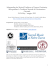### ACHA spring meeting schedule - Cushwa Center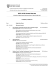### RECENT ADVANCES in MATHEMATICAL and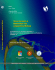### Computational Aeroelasticity with CFD models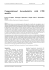### Full text - Dipartimento di Scienze della Terra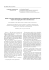### recibido 23 nov 2d15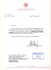### Title list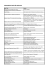### Division I